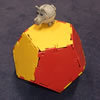#### You may also like### Proximity

We are given a regular icosahedron having three red vertices. Show that it has a vertex that has at least two red neighbours.### Platonic Planet

Glarsynost lives on a planet whose shape is that of a perfect regular dodecahedron. Can you describe the shortest journey she can make to ensure that she will see every part of the planet?### Three Cubes

Can you work out the dimensions of the three cubes?

# Sliced

##### Age 14 to 16 Challenge Level:

Daniel, from Wales High School, sent us this very elegant solution:

The formula for the volume of a tetrahedron is $$1/3 \times \text{area of base} \times \text{perpendicular height}$$ If you slice the tetrahedron in half through $b$ you end up with two equal smaller pyramids. I will work out the volume of one and then multiply by $2$ because they have equal volumes. So to start, I must work out the area of the base. The area of a triangle is calculated as follows: $$1/2 \times \text{base} \times \text{perpendicular height}$$ The base is $a$ and the perpendicular height is $b$ so the area of the base is $$(1/2)\times a\times b=(a b/2)$$ So using the formula for the volume of a pyramid: $$\text{Volume}=(1/3) \times (ab/2)\times (a/2)=a^2b/12$$ Times this by $2$ to get the volume for the big tetrahedron: $$(a^2b/12)\times 2=2a^2b/12 =a^2b/6$$ So the volume of the big tetrahedron is $a^2b/6$.